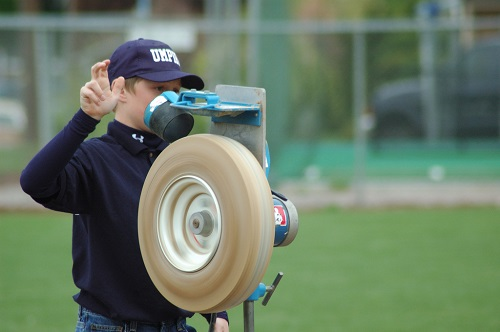# 12.2: Fixed-Axis Rotation

$$\newcommand{\vecs}{\overset { \rightharpoonup} {\mathbf{#1}} }$$ $$\newcommand{\vecd}{\overset{-\!-\!\rightharpoonup}{\vphantom{a}\smash {#1}}}$$$$\newcommand{\id}{\mathrm{id}}$$ $$\newcommand{\Span}{\mathrm{span}}$$ $$\newcommand{\kernel}{\mathrm{null}\,}$$ $$\newcommand{\range}{\mathrm{range}\,}$$ $$\newcommand{\RealPart}{\mathrm{Re}}$$ $$\newcommand{\ImaginaryPart}{\mathrm{Im}}$$ $$\newcommand{\Argument}{\mathrm{Arg}}$$ $$\newcommand{\norm}{\| #1 \|}$$ $$\newcommand{\inner}{\langle #1, #2 \rangle}$$ $$\newcommand{\Span}{\mathrm{span}}$$ $$\newcommand{\id}{\mathrm{id}}$$ $$\newcommand{\Span}{\mathrm{span}}$$ $$\newcommand{\kernel}{\mathrm{null}\,}$$ $$\newcommand{\range}{\mathrm{range}\,}$$ $$\newcommand{\RealPart}{\mathrm{Re}}$$ $$\newcommand{\ImaginaryPart}{\mathrm{Im}}$$ $$\newcommand{\Argument}{\mathrm{Arg}}$$ $$\newcommand{\norm}{\| #1 \|}$$ $$\newcommand{\inner}{\langle #1, #2 \rangle}$$ $$\newcommand{\Span}{\mathrm{span}}$$$$\newcommand{\AA}{\unicode[.8,0]{x212B}}$$

With rigid bodies, we have to examine moments and at least the possibility of rotation along with the forces and accelerations we examined with particles. Some rigid bodies will translate but not rotate (translational systems), some will rotate but not translate (fixed axis rotation), and some will rotate and translate (general planar motion). Here we will examine rigid body rotation about a fixed axis. As the name would suggest, fixed axis rotation is the analysis of any rigid body that rotates about some axis that does not move. Many devices rotate about their center, though the objects do not need to rotate about their center point for this analysis to work.Figure $$\PageIndex{1}$$: The wheel on this pitching machine is an example of fixed axis rotation with the axis of rotation at the center of mass. Image by Abigor, license CC-BY 2.0.

We will again start with Newton's Second Law. Since this is a rigid body system, we include both the translational and rotational versions.

$\sum \vec{F} = m * \vec{a}$

$\sum \vec{M} = I * \vec{\alpha}$

By setting up free body diagrams, determining the equations of motion using Newton's Second Law, and solving for the unknowns, we can find forces based on the accelerations or vice versa.

## Balanced Rotation

If the center of mass of the body is at the axis of rotation, which is known as balanced rotation, then acceleration at that point will be equal to zero. The pitching machine above is an example of a balanced rotation, and most fixed axis systems will be intentionally built to be balanced. With the acceleration of the center of mass being zero, the sum of the forces in both the $$x$$ and $$y$$ directions must be also be equal to zero.

$\sum F_x = 0$

$\sum F_y = 0$

In addition to the force equations, we will can also use the moment equations to solve for unknowns. In simple planar motion, this will be a single moment equation which we take about the axis of rotation or center of mass (remember that they are the same point in balanced rotation).

$\sum M_O = I_O * \alpha$

## Unbalanced Rotation

When the center of mass is not located on the axis of rotation, the center of mass will be accelerating and therefore forces will be exerted to cause that acceleration. In perfectly anchored systems these will be forces exerted by the bearings, though these forces can often be felt as vibrations in real systems.Figure $$\PageIndex{2}$$: Many video game controllers use motors to rotate the small masses where the center of mass is not at the axis of rotation. This unbalanced rotation results in forces (felt as vibrations) needed to accelerate the center of mass of the spinning masses. Image by unknown author under a CC0 license.

The kinematics equations discussed in the previous chapter can be used to determine the acceleration of a point on a rotating body, that point being the center of mass in this case. After determining those accelerations, they can be put into force equations, most likely using the $$r$$ and $$\theta$$ directions.

$\sum F_r = m a_r$

$\sum F_{\theta} = m a_{\theta}$

Note that as the body rotates, the direction of the acceleration and the direction of the forces change. Also note that the further the center of mass is from the axis of rotation, the larger the mass. The larger the angular velocity, the larger these forces will be.

To supplement the force equations, we can use a moment equation about either the axis of rotation or the center of mass, as these are no longer the same point. Whichever is chosen, just be sure to be consistent in taking the moments and the mass moment of inertia about the same point.

$\sum M_O = I_O * \alpha \quad\quad \text{or} \quad\quad \sum M_G = I_G * \alpha$

More information on how to calculate the mass moment of inertia for a body can be found in Appendix 2.

Example $$\PageIndex{1}$$

A hard drive platter 8 cm in diameter accelerates at a constant rate of 150 rad/s2. If the hard drive weighs a uniformly distributed 0.05 kg and we approximate the hard drive as a flat circular disc, what moment does the motor need to exert to accelerate the drive at this rate?Figure $$\PageIndex{3}$$: A picture of a hard drive that emphasizes the flat circular platter.
Solution

Example $$\PageIndex{2}$$

The drum in a washing machine can be approximated as a cylinder 0.4 meters in diameter and 0.3 meters in height with a uniformly distributed mass of 35 kilograms when full. If we wish to achieve an acceleration of 15 rad/s2, what torque must the motor exert at the center of the drum?Figure $$\PageIndex{4}$$: problem diagram for Example $$\PageIndex{2}$$. A front-loading washing machine, and an approximation of the machine's drum as a cylinder rotated about its axis by the motor.
Solution

This page titled 12.2: Fixed-Axis Rotation is shared under a CC BY-SA 4.0 license and was authored, remixed, and/or curated by Jacob Moore & Contributors (Mechanics Map) via source content that was edited to the style and standards of the LibreTexts platform; a detailed edit history is available upon request.